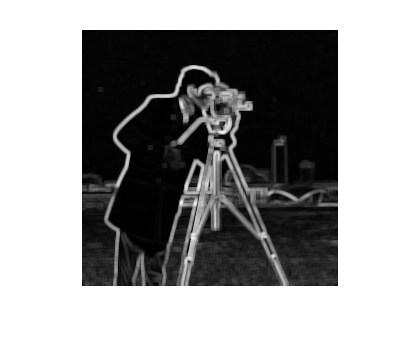# Nonlinear operations using imfilter

Some nonlinear image processing operations can be expressed in terms of linear filtering. When this is true, it often provides a recipe for a speedy MATLAB implementation. Today I'll show two examples: Computing the local standard deviation, and computing the local geometric mean.

The local standard deviation operator is often used as a measure of "busy-ness" throughout the image. For each pixel, the standard deviation of that pixel's neighbors is computed:

sqrt( sum ( (x - xbar).^2 ) )

(Scale factors omitted.)

Mathematically, the summation can be rewritten as:

sum(x.^2) - sum(x).^2/N

Both of these local sums can be computed by using imfilter with an all-ones filter.

I = im2double(imread('cameraman.tif'));
imshow(I)Original image credit: Massachusetts Institute of Technology

To compute the sum(x.^2) term, we square (elementwise) the input to imfilter.

h = ones(5,5);
term1 = imfilter(I.^2, h);
imshow(term1, [])Notice the dark band around the edge. This is because imfilter zero-pads by default. We might want to use the 'symmetric' option instead.

term1 = imfilter(I.^2, h, 'symmetric');
imshow(term1, [])To compute the sum(x).^2 term, we square the output of imfilter.

term2 = imfilter(I, h, 'symmetric').^2 / numel(h);
imshow(term2, [])Then we subtract the second term from the first and take the square root.

local_std = sqrt(term1 - term2);  % scale factor omitted
imshow(local_std, [])Cautionary notes

• The procedure shown here is not always a numerically sound way to compute the standard deviation, because it can suffer from both overflow (squared terms) and underflow (cancellation in the subtraction of large numbers). For typical image pixel values and window sizes, though, it works reasonably well.
• Round-off error in the computation of term1 and term2 can sometimes make (term1 - term2) go slightly negative, resulting in complex outputs from square root operator. Avoid this problem by using this code:
local_std = sqrt(max(term1 - term2, 0));

Recent releases of the Image Processing Toolbox include the function stdfilt, which does all this work for you.

The local geometric mean filter multiplies together all the pixel values in the neighborhood and then takes the N-th root, where N is the number of pixels in the neighborhood. The geometric mean filter is said to be slightly better than the arithmetic mean at preserving image detail.

Use the old logarithm trick to express the geometric mean in terms of a summation. Then imfilter can be used to compute the neighborhood summation, like this.

geo_mean = imfilter(log(I), h, 'replicate');
geo_mean = exp(geo_mean);
geo_mean = geo_mean .^ (1/numel(h));

imshow(geo_mean, [])Does anyone have other creative uses of imfilter to share?